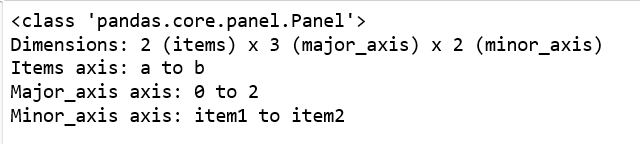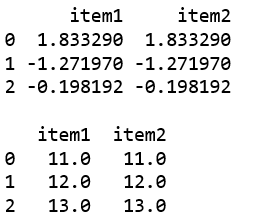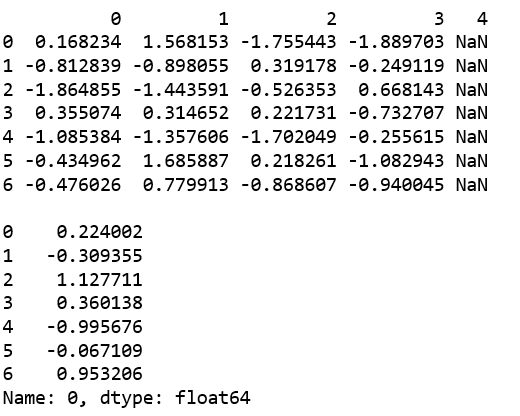Python | Pandas Panel.clip_lower()

In Pandas, Panel is a very important container for three-dimensional data. The names for the 3 axes are intended to give some semantic meaning to describing operations involving panel data and, in particular, econometric analysis of panel data.

Panel.clip_lower() function is used to return copy of the input with values below a threshold truncated.

Syntax: Panel.clip_lower(threshold, axis=None, inplace=False)

Parameters:
threshold : Minimum value allowed. All values below threshold will be set to this value.
float : every value is compared to threshold.
array-like : The shape of threshold should match the object it’s compared to.
axis : Align self with threshold along the given axis.
inplace : Whether to perform the operation in place on the data.

Returns: same type as input.

Code #1: Creating a Panel using from_dict()

 # importing pandas module  import pandas as pd  import numpy as np    df1 = pd.DataFrame({'a': ['Geeks', 'For', 'geeks'],                      'b': np.random.randn(3)})                        data = {'item1':df1, 'item2':df1}    # creating Panel  panel = pd.Panel.from_dict(data, orient ='minor') print(panel, "\n")

Output:Code #2: Using clip_lower()

 # importing pandas module  import pandas as pd  import numpy as np    df1 = pd.DataFrame({'a': ['Geeks', 'For', 'geeks'],                      'b': np.random.randn(3)})                        data = {'item1':df1, 'item2':df1}    # creating Panel  panel = pd.Panel.from_dict(data, orient ='minor') print(panel, "\n") print(panel['b'], '\n')       df2 = pd.DataFrame({'b': [11, 12, 13]}) print(panel['b'].clip_lower(df2['b'], axis = 0))

Output:Code #3:

 # creating an empty panel import pandas as pd import numpy as np    data = {'Item1' : pd.DataFrame(np.random.randn(7, 4)),          'Item2' : pd.DataFrame(np.random.randn(4, 5))}            pen = pd.Panel(data) print(pen['Item1'], '\n')    p = pen['Item1'].clip_lower(np.random.randn(7)) print(p)

Output:My Personal Notes arrow_drop_upCheck out this Author's contributed articles.

If you like GeeksforGeeks and would like to contribute, you can also write an article using contribute.geeksforgeeks.org or mail your article to contribute@geeksforgeeks.org. See your article appearing on the GeeksforGeeks main page and help other Geeks.

Please Improve this article if you find anything incorrect by clicking on the "Improve Article" button below.

Article Tags :

Be the First to upvote.

Please write to us at contribute@geeksforgeeks.org to report any issue with the above content.